Select Page

### The Earth is Round

“Know you not? hear you not? Has it not been told you from the beginning? Have you not understood the foundations of the earth?”

– Isaiah 40:21

“It is He that sits above the circle of the earth, and the inhabitants thereof are as grasshoppers; that stretches out the heavens as a curtain, and spreads them out as a tent to dwell in;”   – Isaiah 40:22First, this was written down by the prophet Isaiah around 700 BCE, about 2200 years before Columbus proved that the world was round.  If the Creator could sit on the circumference, then the earth was a sphere. Next, the image of the heavens stretching out like a curtain is reminiscent of the endless fields that both the ancient kabbalists and the modern physicists describe. The tent is akin to a scaffolding, possibly the Essential Cube of Creation. “Have you not understood the foundations of the earth?” For anyone that has not been following this series from its inception, you can find the entire work here.

As told to you from the beginning, Torah’s description of Creation begins with a virtual earth without form or substance. “With darkness on the face of the depths.” Darkness has a complete gematria of 378, as in the value of the 27 ordinal positions.  G-d then creates Light (Flow) and water (Fields). Then He puts a firmament in the middle of the water (Fields) and the water (Fields) separated into above and below. Can the firmament (רקיע) be the central 14 position in the Essential Cube of 27 positions (fields)? And the separation the two directions (spins) of the Flow, upward and downward?

The firmament is known kabbalistically and spiritually as a restrictive barrier. Its gematria of 380 is the same as Mizraim, a restrictive passage or strait, that all the positions must pass through, hence the shape of the letter Nun (נ) that occupies that position. 26 positions, split in 13 (אחד) above and 13 (אחד) below, One that is One and One, that become 42 when they pass through.

“G-d named the firmament (רקיע) Heaven (שמים)” with the common letter, yud (י) of numerical value 10, as in the numerical difference between the two words, (390380) = 10. Once the spin was set, the Flow through the 27 positions of the Cube created the 10 loops or sefirot.

“And God said: ‘Let the waters under the heaven be gathered together unto one place, and let the dry land appear.’ And it was so.” – Bereshit 1:9

It was then that the Fields could be gathered to one place, as proscribed in the 9th verse. And in the 10th verse He named that creation called dry land and what we call physicality, Earth. In was in verse 9 that the laws of physics could take hold and develop.

Physicists talk about the fundamental particles, but the truth is that most now believe that they are really fields, specific perturbations or excitations in the fields, one specific field for each particle. For example, they view the electron as a .511 MeV perturbation in the electron field. This is called the resting mass of the field.  A perturbation or oscillation of any other magnitude does not create an electron, or anything else, in this particular field.

We think of particles as round and free to move around, yet their quantum field definition reads more like a protuberance that is confined to the field of which it is constructed, like a specific wave in the ocean. In fact, they are considered quantized waves. It is called quantum field theory(QFT). Physicists believe there are 17 of these fields plus another field that must be there for the alleged graviton, making 18 in total.  Of course, the physicists do not have an explanation for what generates them, and they do suspect there must be more fundamental fields underlaying these 18. We can add the 4 energy movements—radial movement, circular movement, counterspatial movement and spatial movement—possibly wrapped in a single field, and the Dielectric and Magnetic fields, together with the plane of Inertia, making 22. We can surmise that there must be 5 higher dimensional fields that give way to all the rest. Can those 5 fields be the 5 Essential elements of Creation, making 27?

The particles exist as 3-d waves in the fields only when the right amount of energy is present and maintained. The fields however are always there and are endless.

The fields can interact with one another, transferring and sharing energy, and even creating temporary (fleeting) anti-particles that cancel out some of the regular particle waves, as in aligning a trough with a wave crest.

The belief in fields means physicists inadvertently believe in the endless and in Oneness. What happens somewhere can affect everywhere when everything is connected.  It is easier to perceive an unseen field than an unseen force. It is also a lot less frightening.

# The Sphere

The earth is a sphere. It was in the days of Isaiah and it still is today. So is the Sun and so is the Moon.  The Essential Cube of Creation can be one as well.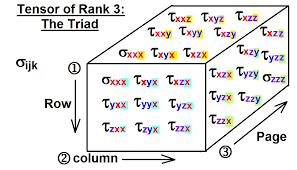Thanks to Stress Tensors, the 27 positions in the Cube can easily be stretched into a Sphere by multiplying it by a 3 x 3 matrix.  It is more advanced mathematics so we will leave it to the mathematicians and move on to tackle more esoteric and abstract matters.

We have already analyzed how the universal harmonic ratio 1.273 converts a square to a circle. In terms of the cube, the ratio of a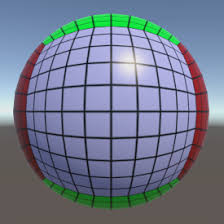side of a cube to the radius of a sphere of equal volume is .620389505, which breaks down to 620 (keter) and 389505, which in turn is 1120 less than the 390,625 elements of the Torah (6252), H’keter squared. Moreover, 1120 is the base 3 number for 42, so it is sort of a backdoor entrance, binding the sphere to the cube.  Please note that more accurately, to 9 decimal places, the ratio works out to .620350491

### Tuck and Roll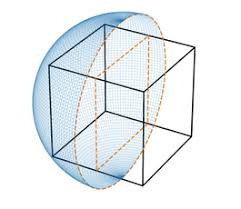That just equates the volume of a sphere with that of a cube.  What we really want to do is envelope or wrap the sphere around the existing Cube, circumscribing it in a force field.

“…stretches out the heavens like a curtain, and spreads them in a tent…”

The tuck and roll, or force field protection from comic book lore, brings a wave of light out of the fields that engulfs the cube oranything else, individually and protects it from the deluge.  Like the ark that protected Noach and the animals from the Flood (the inundation of the fields), a bubble is formed. The tuck and roll process avoids the fields, transforms cubes to spheres and encases them in round force fields. Examples of this process when the right kavannot are utilized, are the Clouds of Glory, the sukkah, the mikveh, and the tallit.

The process also forms a tube or tunnel.

### The Time BubbleWe are already encased in one such spherical bubble, time. The kabbalists looked on this bubble as a protection against the laws (fields) of nature.  By giving us time, the results of cause and effect are dampened, and space is provided for us to make corrections and better choices.  In the time bubble, time starts in the center, not at one end of a line.

We are already in the event horizon. We are already at the edge of the bubble where cause and effect are one, where our foul feelings come flooding out of our mouths and our finger tips, and even our thoughts have consequences, and worse.

### The Cube in the Sphere

Let us review.  In the Essential Cube of Creation based on the number and Name of 42, there are 27 numbered positions from 127, arranged is a very particular order, with the position 14 in the center.

Each of the 27 numbers matches a different ordinal value for each of the 27 Hebrew letters, which total 378 for the 27 ordinal values and 4995 for the 27 actual letter values. Altogether, (27 + 378 + 4995) = 5400. Moreover, the sum of the digits in (27 + 378 + 4995) = 54, the higher dimensional reason that there are 54 portions in the Torah.

It can be said that the 42-Letter Name field led to the creation of the Heavens, and the Bereshit Field to the Earth. Combined (2701 + 3701); they led to Heaven and Earth, to Binah (1000) hyperspace + the (2) opposing spins and the Essential Cube (5400) od Creation. Together (6402), they led to the central positional cube of 14, which has a complete gematria of 64.

What is the Essential Cube of Creation? All 27 fundamental fields intwined in One and integrated around the primordial Flow of the 10 sefirot. Why is the value of Bereshit 2701 and that of the 42-Letter Name 3701? Because Bereshit connects with Creation and the 27 fundamental fields, and the 42-Letter Name connects to those 27 fundamental fields of the Essential Cube plus the Flow of 10 loops (sefirot).When the Cube is protectively encased in a Sphere, and the volume of the Cube is One(1) the resultant volume of that Sphere is necessarily 2.7207, reflecting the 27 fundamental fields intwined in One, and the Light (207).  Just to let us know that we were on the right track, the Creator arranged it so that the volume of the spheres that encased Cube of side 1 plus an encased Cube of side 2 together would equal 24.48…, as in the year 2448 HC when we received the Torah at Mt Sinai, a cosmic moment when we were encased in the Sphere of Protection for a 2nd time. This exact moment was 42.4% of the way to the event horizon. Or even more precisely, it is φ3 % of the way, a ratio of Phi(φ) which was integral to the spiraling vortexes of magnetic radiation, among many other phenomena that we now view as fields.

He also knew that the solar rotation (sidereal) period would be about 24.48 days at the Sun’s equator, and that the sum of logs of bases 1 – 10 for the number 54 is 24.48. Because of how the formulas work out, the sum of the volumes for all the spheres circumscribing cubes of sides (124 units) is 105 times that of 24.48 or 244862.91. By the way, 291 is the numerical value of earth in Hebrew.  Incidentally that 24thSphere has a volume of 37610…. Is that indicative of1313years later that2448 HC, the year 3761, or year 1 CE in the western calendar with 1.313 being the exponent coefficient of the Alef-bet? Given that 24 hours in a day is a major component in our reference of time, there may indeed be a strong correlation and a higher dimensional reason for these relationships with time. Moreover, the sum of the volume of all 24 of those respective inscribed cubes is 90,000.

Moreover, the sum of the volumes for all the spheres circumscribing cubes of sides (14) is 102 that of 2.7207 or 272.07. Four Essential Elements, each their own cube encased in a sphere, equals 1 Cube of One, expanded 100-fold.  These last two expansions are based on the higher dimensional sum of integers, in that the sum of (1 – 4) integers is 10 and 10 squared is 102, while 24.48 represented the sum of (1 – 2) or 3 and the sum of the integers (1 – 24) is 300, which is 100 x 3 and 100 squared is  105.

Using the central 14 position of the Essential Cube of Creation as a mid or central summation point, the sum of the volumes for all the spheres circumscribing cubes of sides (114) is 29995.707, or about (30,000 5).  The 5707 portion is the number of years before Israel became a nation and also the ratio of the area of a circle that circumscribes a square, Pi/2 or 1.5707.  Also, since the outer portion of the circle is 27.3% of the total area of the circle, the square is .5707 or (57.07%) of the circle.  When the One sat on the circle of the earth He knew it would take 5707 years for Israel to became a nation (1948 CE), and that the ratio of the darkness (הַחשֶׁךְ) to the light(הָאוֹר), 333/212 equals 1.5707.

We can be forgiven for thinking that there must be a causation effect since we were dealing with a sphere circumscribing a cube and there well may be, but it is not so simple as we added 14 of those spheres together.  On the other hand, 14 is the gematria of David, whose life marked the midpoint toward the already stretched thin event horizon of the time bubble.

Was He sitting on the circle of Earth or on the Sphere of time, the tent in which we dwell?

The first 14 ordinal values of the 27 in the Essential Cube of Creation gave us 14 spheres that totaled 29995.707 and final (1527) yields a sum of 358748.6…

# The Universal Harmonic

### Five (5)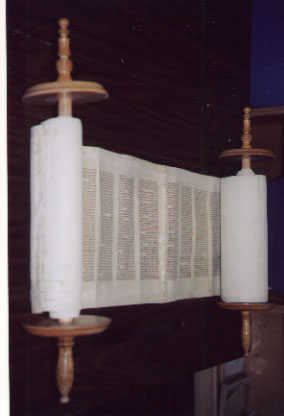The total gematria of the Torah is 21009826 and that of the 72 Triplets is 9143. Subtracting the 72 Triplets from that total, and also subtracting the first and only independent and explicit Triplet of the 15 Triplets of the Shema (שמע), 410, we get a resultant value of 21000273 for the Torah,-+ net of those 73 Triplets, as in the 73 times that the Torah’s 5th word, “(השמים), the Heavens,” reoccurs in the Torah.

The value 410 is also the number of years the First Holy Temple stood on earth.

The Heavens, the Torah’s 5th word, as distinguished from the earth, can be said to represent the 5 Essential Elements created before everything else.   One of the ways this plays out with the use of higher dimensional mathematics and its intersection with Time is found in the sum of the first Triplets in each of the 5 Essential Elements of Creation (6 + 203 + 410 + 1143 + 14) which equals 1776.  Please note that we used 1143 as the first row of the 72 Triplets rather than the first of the Triplets. You may have noticed also that the first Triplets of Bereshit and Shema sum to 613, as in the 613 mitzvot, and that the first Triplet of the Shema and the first position of the Essential Cube (410 + 14) = 424, the numerical value of Moshiach Ben David.

There are 42 4-digit sequences repeated within the first 1000 digits of Pi and only one 5-digit repeated sequence, 42019. In fact, it is found at digit #702, as in Shabbat of numerical value 702, and again at the end the 1000 digits with the sequence (3.14…216420198). As it happens, the total gematria of the Torah (21009826) divided by 5 equals 4201965.

### 21009826/5 = 4201965

This resultant 21000273 from above brings us back to the universal harmonic, 1.273.  We see it encoded in the Torah via the total of the 5 elements: 401273. We see it in the Pyramid with the ratio of its height to half its base: 210/(330/2) = 1.273. We see it in the solar system with the ratio of the size of the Earth to the (Earth and Moon) combined: 1 : 1.273; and through the ratio of the Sun to Earth: (4 x 27.3) : 1; and through the ratio of the Sun to the (Earth and Moon) combined: (27.3 x Pi) : 1.  We see it in the temperature scale of the universe through absolute zero, -273o K. We see it in the physical design of our reality in that 4/Pi = 1.273… and (4 Pi)/Pi = 0.273….  and in the relationship of the 4-sided square to the inscribed circle 1.273 : 1.

As Peter pointed out, we also see it in the placement of 1273 within Pi, immediately after digit #296, which is the gematria of “earth” the 7th and last word of the Torah’s first verse. Even more interesting is that the actual sequence that starts at digit #296 is …41273…, as in 401273 elements. The next time we find 1273 in Pi is at digit #14404 and it immediately follows 296: …2961273

This circles back to “the One who sits on the circumference of the earth” and to the 365 times that the Torah’s 1st word(בראשית), In the Beginning” and its 7th word “(הארץ) earth” collectively reoccur in the Torah, given that the earth orbits the Sun every 365 days.

We also see this universal harmonic in the 4 Essential Elements of Creation, the 112 Triplets, whose initials of the 11 Triplets of Bereshit together with the initials of the 15 Triplets from the Shema total 1273.  The 26 initials of these 26 Triplets—26 being the numerical value of the Name of G-d (יהוה)—were designed to equal 1273, the universal value that squares the circle, defines our solar system, and is built into the Phi(φ) defined dimensions of the Torah (401273 entities).

### The Phi(φ) Spiral and 42-Field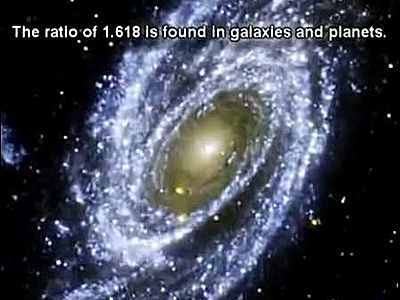By the way, while we know those 401273 elements of the Torah equal 248,000 times Phi(φ), it is significant to note that 181.8181 times Phi(φ)15 = 248,000.0, meaning 181.8181 x φ16 = 401273 too. The significance is found in that the sum of the 3 iteration of Alef(א) = 1818; therefore, both 248,000 (and consequently 248) and 401273 can be said to be derivative of Alef(א).

By the way, 181.818181820, as in the ordinal values (18 and 20) of (רצ) at the center of the 42-Letter Name, equals 2750363.6…, or the cubit (27.50) and H’Moshiach (363). Why is it significant that (רצ) or 18 x Phi(φ)20 is at the center of 42? We must recall that Phi(φ) is a path along a spiral.  The spiral begins with Alef(א), the One, and passes through 248000 and 401273—the intertwined Torah, universal harmonic, and E8 Lattice—along the way, then through the a point containing the cubit (27.5”) and H’Moshiach (363). We already know 42 is in the center of the center of the universe.  Is this primordial path meant for us to follow?

The Hezbollah connections aside, is it a synchronicity, or coincidence, that 2750 tons of ammonium nitrate exploded this week?

Or the 112-mph derecho that swept through Ohio this week? Or the Abraham (248) Accords signed this week?

In the previous chapter, we discussed the value 60 of the letter Samech (ס)and that there are 1833 letter Samechs (ס) in the Torah and that the value of the 42-Letter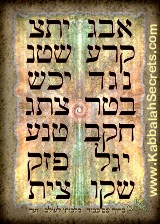Name through its central midpoint is 1833.  That central midpoint is found right between the central letter pair (רצ) of the 42-Letter Name, as in the equation 18 x Phi(φ)20 and the 3 iterations of Alef(א), 1818   As it happens, (181.8181818/3) = 60.60606060

And given the relationship between (רצ) and Phi(φ) is it odd that the Torah specifically mentioned the Flood occurred in the 601st year of Noach’s life and that at digit #601 in Pi we have the numeric string …2000… and that from that point on till the end of the 1000 digits, the remaining digits sum to 1800.

### Harmonics in the Torah and its First verse

Speaking of the 26 initials, we see the universal harmonic 1273 in a triangular relationship between the Torah’s first verse, Moses, and Phi(φ)2, or 2.6180…, in that (2.61801.273) = .3450.  The 4th (את) and 6th (ואת) words of the Torah collectively reoccur 3450 times in the Torah or (10 x 345), the gematria of Moses (משה). While these initials (משה) also comprise the 5th Triplet of the 72 Triplets and the Torah is known as the 5 Books of Moses, it should be noted that there are 5 letters in the 4th (את) and 6th (ואת) words and that (4 + 6) = 10. This was in the same way that the 1st word (בראשית) and the 7th word (הארץ) have 10 letters, and that (1 + 7) = 8.

By the way the 3rd word Elohim(אלהים) is found 228 times in the Torah, so when we collectively combine the recurrences of the 3rd word, the 5th word, and the 7th and 1st words (365), we get (228 + 73 + 365) = 666.  Notable is that 3571 is φ17 or 5778/φ. And like Glen pointed out, the sum of the squares of the first seven primes, through 17: (22 + 32 + 52 + 72 + 112 + 132 + 172) = 666.

We have seen numerous connections to 21009826, the total gematria of the Torah, yet another is found in the sum of the 14 square roots of the 14 Triplets of the 42-Letter Name: 210.0262873… While 21002628 is evocative of 21009826, off of it by 7200 (including the kolel), the number 2628 is the sum of the Torah reoccurrences of the Torah’s 4th (את) and 2nd (ברא) words.  It is also the sum of the integers from 1 – 72. Moreover, since we know the Torah’s 5th word (השמים), Heaven, reoccurs 73 times in the Torah, the total recurrences of the 4th, 2nd, and 5th words together is (2628 + 73) = 2701, the value of the Torah’s first verse.

Earlier in this series, we divided the 5 Essential Elements into natural splits of energetically male and female components (1143 + 302 + 1000 + 410 + 1) = 2856, which aligned with the total words in the Torah divided by 28, or 2856.2856 and with the 28056 letters Hei(ה) in the Torah, each of numerical value 5.  Splitting that ratio of male/female for the 5 Elements of 2856/14136 equaled 20.20373, as in the year 2020 CE, the 203 value of (ברא), the Torah’s 2nd word, and the 73 reoccurrences of the Torah’s 5th word (השמים).  What is most germane is that the Torah reoccurrences of the 2nd, 3rd, and 4th words together also equal 2856.

### The Universal Harmonic in the Names

The universal harmonic also appears within the 14 Triplets of the 42-Letter Name in the differential between the 6th Triplet (330) and the 5th (57) Triplet, as in (33057) = 273.

We also see it in the full gematria value of the phrase I Am that I Am (אהיה־אשר־אהיה), that is equivalent to 546 when the kolel of the 3 words is included, which in turn is identical to that of the sum of the initials of the 10 sefirot/dimensions (כחבחגתנהימ), whose small gematria sums to 42. The value 546/2 = 273. The same holds true for the Name, Israel (ישראל), whose numerical value with the kolel for its 5 letters is 546, as explained by the Arizal.

The 26 initials of these 26 Triplets that equal 1273 are on one axis of the Primordial Alef from which the 112 Triplets emanate. On the other axis are the 86 Triplets of 42-Letter Name and the 72 Names (Triplets) combined. Together, their initials total 3842, the exact same value as first 42-letters of the Torah that are associated with the origin of the 42-Letter Name. They are also equivalent to the first 21 spelled-out letters of the Alef-bet. No one in their right mind would think this coincidence, so let us explore further.

By the way, this is also 280 less than the collective 4122 reoccurrences of all 7 words of the Torah’s first verse in that (41223842) = 280, the numerical value of the 5 final letters (MaNZiPaC) and the ordinal value of the Torah’s first 27 letters.

When the 42-Letter Name was transposed to the first 42 letters of the Torah, there was a letter purposely avoided, a specific letter Hei(ה) of value 5, as in the 5 Essential Elements.  The total value for all 28056 letters Hei(ה) in the Torah is 140,280, like the 140 and 140 split and 280 total ordinal value of the Torah’s first 27 letters and like the position number 14 at the center of the 5 Essential Elements, whose value is 50, like the Gates in Holy Temple that were 50 cubits high and 14 feet thick.

### The Priestly Blessing

The priestly blessing is found in the Torah in paragraphs 38-42 of Bamidbar, chapter 6 verses 2227. In the Zohar, regarding parsha Eikev 3:40, it is stated, “Three times fourteen equals 42, and the three Yuds in three times hand (יד) are indicated in “Hashem bless you, Hashem make His face shine upon you…Hashem light up His countenance to you” of YHVH, YHVH, YHVH [from the priestly Blessing]. The three Dalets in three hands (יד) are implied in Adonai, Adonai, Adonai. The prophet said about them, “The Temple of Hashem, the Temple of Hashem, the Temple of Hashem, are these” The value of the YHVH along with the implied Adonai is 91 and thus the 3 of them equal 273. “[The priests] will thus link My Name with the Israelites and I will bless them.”

Understanding begets understanding.

### The Cubit

The ratio between the 112 Triplets split along these two axes of the Alef(א) is thus 3842/1273 = 3.018, which is precisely equal to the cube root of 1 cubit: 3√27.5 = 3.018 = 3842/1273. So, if we cube the ratio (3842/1273 = 3.018) of the Alef axes formed by the 112 initials of the 4 Essential Elements(Forces) we get 1 cubit.  Four important fields of Creation aligned with 4 Names of G-d create the Cubit. Cube; Cubit.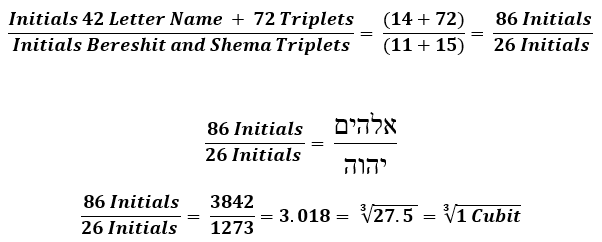The first 42-Letters of the Torah that summed to 3842, as the other part of the 3842/1273 = 327.5 = 3.018 equation, are cosmically conjoined with the 42-Letter Name that comprises the 14 Triplets of the 4 Essential Elements (Fields). Moreover, (1273 + 3842) = 5115 and the sum of the digits in that equation = 42, while 1273/5115 = .248

### Phi, Fibonacci and the Baked-in Cubit

As previously discussed, there is yet another connection between the hidden cubit (27.5”) and Phi(φ). It is well known that the division of any two sequential Fibonacci numbers will tend toward the Phi(φ) ratio.  Viswanath determined the growth rate of the random recurrent Fibonacci sequence to be a constant equal to 1.1319882487943, and it is known as Viswanath’s constant.  Since he knew nothing about the cubit (27.5”) he missed the connection between his constant, Phi(φ), and the cubit.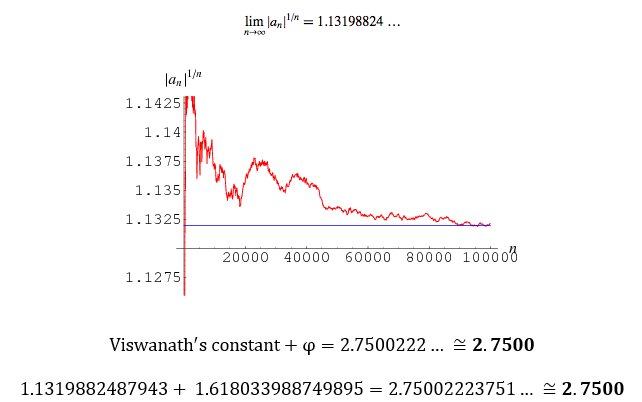# The Spirit on the Waters

“God’s spirit moved on the face of the waters.” What are the waters that existed before light? They are the fields. Spirit (רוח) has a complete gematria of 248. Is this a hint as to how many fields?

We spoke of how higher dimensional numbers are active, not passive, and how certain mathematical interactions between them are reflective of specific patterns we observe in our dimension. We did not explain how those interactions might work, only how to observe them as windows into higher dimension processes and motives. We do not yet know how numbers can attract or how they synchronize.  But….

### Unity

What if numbers are a numeric field, or are contained in one? This would be a field where the excitation or oscillation unit is 1. Thus 1 would be indivisible, in the same way that we view a down quark in a down quark field.  There would not exist a ½ unit, but there could exist multiple single (1) units, like there can be multiple quarks.

Every time 10 units are generated and gathered, they rise up together into a separate, but parallel sub-field, like the electrons in the electron shells in an electron field. The limit for any grouping per field level would be 9.  Hence, the possible sets in any one level would be (1,11,111,1111,11111,111111,1111111,11111111, or 111111111). Each of the units in the 9 numbers would be identical but consist of larger sets from 1 – 9 units each.

The number 111 in our world would be 1 in the primary field, 1 in the secondary field, and 1 in the tertiary field, complex but highly efficient given that these fields are virtually occupying the same space. The number 42 would be 11 in the primary world and 1111 in the secondary field. We could now understand the number 358 as an intercession of the Phi field with the numeric field—11111111 in the Primary field, 11111 in the secondary field and 111 in the tertiary field—rather than as an abstract portal #358 in hyperspace.

We can see the harmony and unity in this, everything based on 1, no “0.”

The number 27 is 1111111 and 11, but the Cube of 27 is unique, as it exists in level One as 3 groups of 9, the limit, but in 3 different axes simultaneously, perpendicular from one another. Nevertheless, they all, 3 x 9, or 27 in total, must equal sets of 42 units each when they are attached, which is still the secret to all the knowledge in the universe.

We ask why there are 42 letters associated with the 42-Letter Name, and 42 Letters of the spelled-out Tetragrammaton in the Upper 42-Letter Name, and why the number of letters has any meaning beyond the letters themselves. It is because the numbers came first. Each letter is assigned to a specific unit (1) in the set of 42 units (111…..1)

The 42 units consist of 8 of the 9 possible unit sets, all except the set of 3: (1,11, 1111,11111,111111,1111111,11111111, or 111111111).

This is also why Alef (א) is 1 and Alef(אלף) is 111, the first of the 3 iterations.

All 9 possible sets (1,11,111,1111,11111,111111,1111111,11111111,111111111) sum to 45, as in the 15 Essential Triplets of the Shema that contain the Upper 42-Letter Name of G-d and total 1118. “The writing is on the wall.” It is also the numerical value (45) of Adam (אדם), whose ordinal value is 18.

As the other fields interact with the numeric field layers, numbers would be recoded that describe the energies or energy levels associated with the processes going on in those fields. When the higher dimensions are imprinted on our world, the place holder “0” is inputed for all the null sets or null layers. For example, the 5 layers  -, 1111, -, 1111, 1 collapse to 14040 in our world.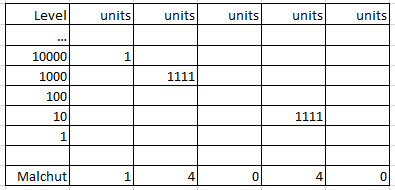Within the endless numeric field, the potential for all numbers exists at all times.  It is a sea of potentiality, not of numerals. The numbers are not sitting out there, waiting for little robots and programs running around to pluck them off Amazon’s shelves. It is the same way that there is not a sea of electrons swimming and swarming, waiting for their chance. The fields are seas of potentiality.

### –Bereshit 1:10

When we see the numbers and physicality in our world, they are no longer potential but fixed. When we break them down, we can see how they were constructed but not what they might have been.  This holds true whether we are speaking of numbers or physical objects. Those objects are eventually made up of molecules, atoms, and quarks but we cannot see them at the field level when they may have combined very differently, forming a very different object, or non-object.

The word the Creator chose for seas (ימים) is very interesting. He specifically named them Yamim (ימים) of numerical value 100, and they are split into (ים) and (ימ) or 5050. The sum of all the integers from 1 – 100 is 5050. And the ordinal value of Yamim (ימים) is 46, as in the Hebrew words Me’ah (מאה), Amah (אמה) and Ha’em(האם), or 100, cubit, and the mother (Binah) respectively.  Binah, as we know, is the hyperspace realm known as the sea of potentiality.  He made the seas complete so they would contain everything, everything in potential.

The earth or physicality (ארץ) He made halfway. From the Alef(א) of the 42-Letter Name to its midpoint (רץ), from 12018. When we examine spherical time and the time bubble this will be an important point.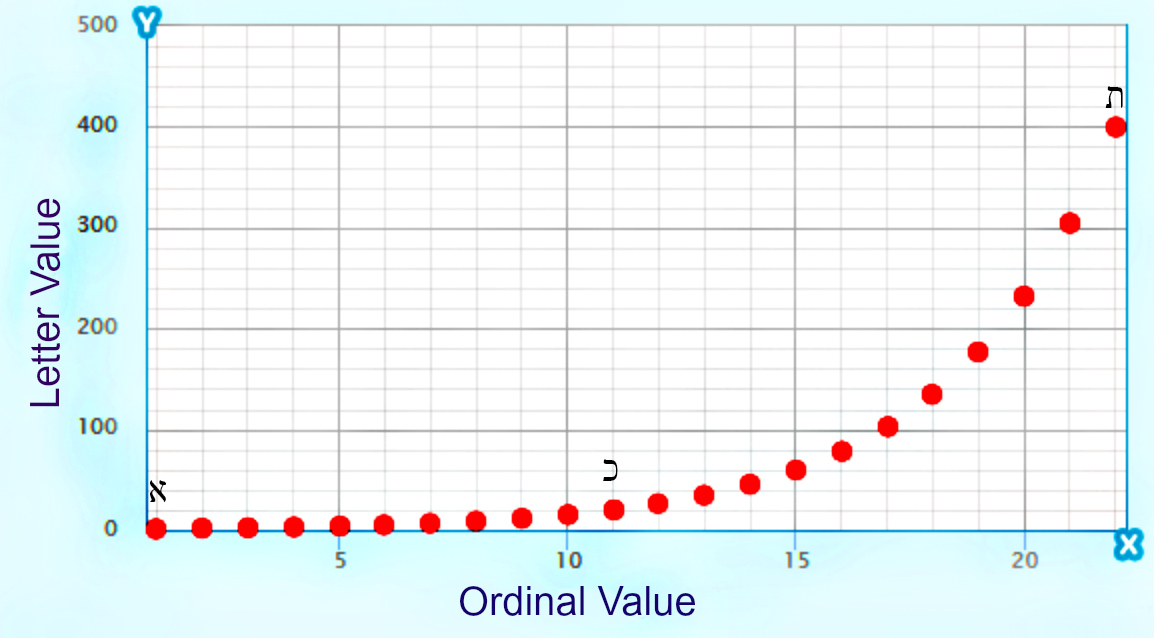Metaphysically, it means He left room for Man to keep creating, to be able to borrow from the seas, potentiality. The last 20 letters of the 42-Letters Name were left to Man to fulfil, from Tav(ת) to Tav(ת). 20 Letters like the 20 levels in the Tower of Truth. From Tav(ת) to Tav(ת), from 202 to 202. The letter Tav(ת) is associated with the end, as in the end of the Alef-bet, yet it is 202 as in Caf(כ)2, as in keter2, the top, head or beginning. Potentiality from beginning to end, like the exponential design of the Alef-bet itself (א־כ־ת).  At every moment Man has the full potential to free himself from the restraints of the physical world or to cement his fate further in physicality. Accepting the linear line of time and death, gives Man no such option.

The numerical value of those last 20 letters is 1778, ultimately splitting 6000 into 1778 and 4000 as Rav Ashlag did. The 222 or Alef(111) x 2 were sacrificed in the .333/.666 light and darkness refining process.  For those that understand, this leaves us with (17782) = 1776 and the P/S cipher for Yamim (ימים) of 1600. The numerical value for Man is 45.

The potential? It is found in the sum of the integers through 291, the numerical value of earth or physicality (ארץ), which is 42486: 424, 4248, 248, and 86, the value of Elohim(אלהים), the Name of G-d associated with the physical world.

### Another Higher Dimensional Math Formula

Number theory is the study of sets of positive whole numbers and the relationships between them. Higher dimensional math is when we use number theory to view what is creating those relationships. While numbers are a series of 1’s in hierarchal fields, their counterparts in our world are base 10 numerals.  As such we can add the digits in those numerals individually or in a series. When we add all the integers from 1 – 42, we are adding the upper level units of each number and get a total of 903 for them. When we add the digits in 1 – 42, we get 273, the universal harmonic.

It all revolves around 42.  Multiply the digits in 903 and we get 27. Multiply them in 27 and we get 14. Multiple the digits in 273 and we get 42.

Likewise, when we sum all the integers from 1 – 36 we get 666. Multiple 6 x 6 x 6 and we get 216, which is equivalent to the sum of all the digits from 1 – 36.

Most relevant to the design of the Cube is that the sum of the digits through number 54 is 378, which in turn is the sum of the numbers from 1 – 27.

The sum of the digits through number 58, which is (10042) is 424, Moshiach Ben David.

Translating that, the Seas of potentiality is split into the two complementary forces 42 and Moshiach Ben David. The value 58 is the quintessential Torah number as 58 and 5/8, and it is also the value of Noach, who was saved from the cleansing (מבול) of the fields in 1658, 120 years before 1778, the age of Man.

“My spirit will not continue to judge man forever, since he is nothing but flesh. His days shall be 120 years.”

The word for that cleaning, mabul (מבול), translated as Flood, begins with 42(מב) and has a gematria of 78, and with an ordinal value of 33 it has a complete gematria of 111, Alef(אלף).

What happened at the end of the age of Man? About 1778 HC we had the Titans and the Tower of Babel? What is happening today, at the end of the 1778-year period delineated above? Why the symmetry?

Time is not linear. It is spherical and begins in the center, not at the edge of a line. Did physicality begin at the edge of a line or did it begin in the center of a sphere?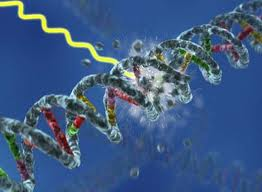Does energy, gravity, etc., radiate spherically from a central point or linearly in a restricted line. Man likes to harness it linearly, in wires, laser beams, etc, but is that allowing it to live up to its full potential? Tesla did not think so. Likewise, Man has put time in a wire, and he even thinks he turned on the circuit.  Beware any vaccine, like mRNA-1273, that injects altered DNA into you. He can turn off that circuit too.

We know why Abraham was giving the gematria 248.  Now we know why Sarah was given the gematria 505.  In the narrative of the Torah, the Creator specifically altered their Names so that we would know their corresponding values were specifically chosen. The age of Man was 120 years. We are specifically told Sarah lived 100 and 20 and 7 years.  She was connected to potentiality as indicated with the 100 and 20, and her ability to give birth without a womb at age 90 (100 for Abraham). She was connected above the Age of Man by 7 years (the 7 sefirot of Zeir Anpin. Sarah lived 127 years. The potential time frame of Man less the universal constant (17781273) = 505. Sarah.

The sum of the integers from 1 – 100 = 5050, potentiality, so fitting because the ordinal value of Sarah(שרה) is 46, that of Me’ah (מאה), meaning 100.

The complete value of Abraham and Sarah together is 840 or (20 x 42).

By the way, mRNA in Hebrew (מרנא) has the same numerical value as earth or physicality (ארץ), the world of limitations.

### Primes

One set of numbers that we look at as indivisible are prime numbers.  These numbers necessarily have no null sets in their bottommost layers.  They may be endless and innumerable, yet they occupy a field of decreasing potentiality, as each successive number cannot be integrally divisible by the previous number or any of the previous numbers before that. Space is a metaphor, but each subsequent prime number has less potential space to occupy than the previous prime.  It is not an actual field but a virtual one, a result of pressure mediations and the pressure of nullity on the numeric field.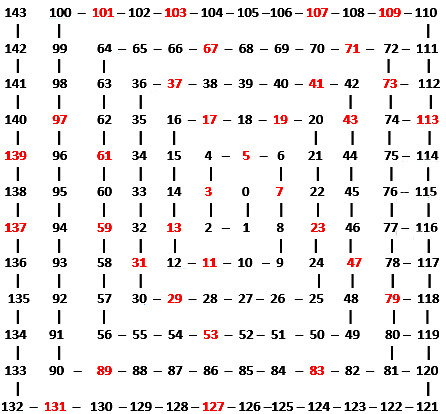Linearly, it is a single long thread, but when a square spiral pressure is applied and the resultant bejeweled numbers wrap around a central starting point, we get a beautiful endless woven fabric whose jewels line up in wonderful diagonal streaks.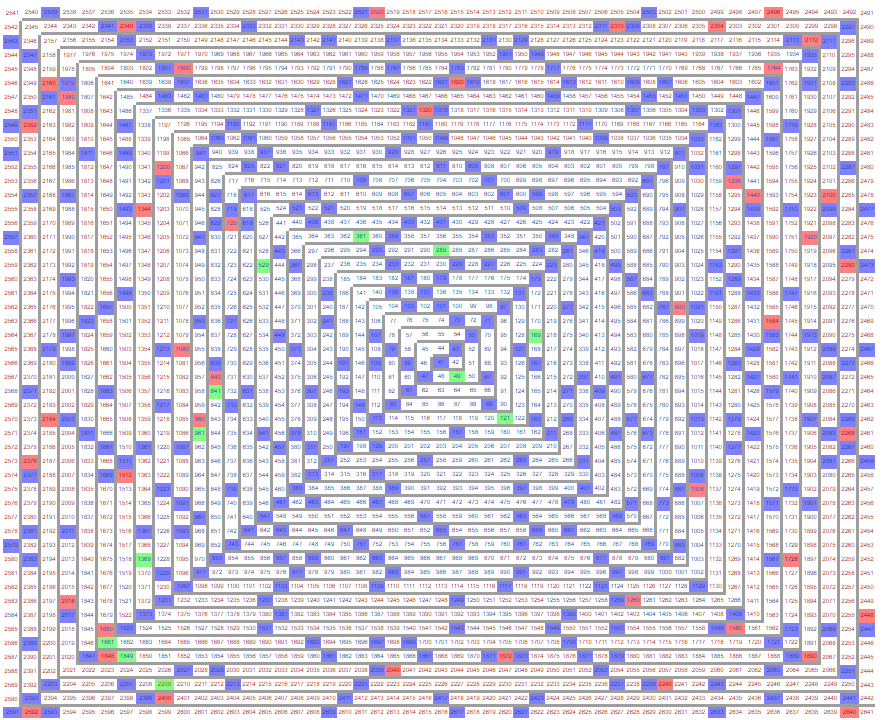Another way to perceive this is as if we are looking through two beautiful layers of woven galactic fabric at a 45o angle from one another, the threads in each are woven perpendicular to one another.Is there something more going on here in higher dimensional space. Maybe, probably. Is there a reason 541, representing Israel is the 100th prime number, or that 613 is the 112th prime, or that 107 is the 28th prime? Surely there is.

Is Israel the 100th prime number because of the connection to the seas of potentiality?

Here is a little cosmic puzzle. We know that summing the integers is a higher dimensional math device used throughout the Torah. The 4th Prime number is 7. The sum of the first 4 primes is 17. The sum of the numbers 17 = 28. (4 x 7) = 28. The 7th Prime number is 17. The sum of the digits from 1 – 17 is 153 and (153 + 17) = 170, and at digit #342 in Pi is the string …17153…. The 28th Prime number is 107.  The sum of the digits through 107th is 5778.  And since 173 is the small gematria of the 42-Letter Name at the center of it all, the puzzle continues with 1/5778 = .0017307026 and the first 173 in Pi is found at digit #428, as in (4 x 28) = 112 Triplets.

“And God called the dry land ‘Earth,’ and the gatherings of the waters He called ‘Seas.’ saw that it was good.”     –Bereshit 1:10

“It is was Good (Ki Tov)” The numerical value for Good(טוב) is 17. While the full value of Ki Tov is 57, its complete value is 95. And while 57 is the complete value of (מב), 42, 95 is the value of “The King (המלך).” He sealed each day with 42 and signed it “The King(המלך).”

### The Names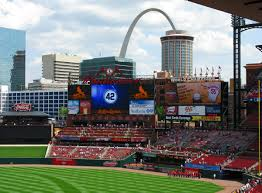But if the water is the fields, what is the Spirit (רוח) moving on the water’s surface. With all 27 or 28 fields set in place and interacting as perceived matter and energy, the entire universe could form, develop, and evolve. But if the Creator wanted to tweak it, improve it, make it trend in specific ways, He would have to intervene, which may or may not be in line with the prime directive. At a minimum, it is inefficient.  Nevertheless, if the Creator wanted to do that, He could accomplish it remotely by utilizing special attractors based on assorted Names of G-d. There could be a separate special field that oscillates in the frequency of those Names, like 14, 42, 21, 26, 65, 72, and 86. This could serve to amplify the effect of the 4 Essential Element Fields.  For example, combinations of these excitations, like (21, 26 and 65), (14, 26 and 72) or (26 and 86) can engage all 112 Triplets, as unifications. These Names could also exist as separate fields onto themselves or sub-fields. If a graviton field could impart gravity to the entire universe in proportion to the particle fields it interacts with and convey that attraction from one end of the universe to the other with no other physical contact between these particles, why could not the Name Fields do the same?

While the Names have values that interact with the numeric fields, etc., these Names are not numbers; they are special attractors or vortices that act as portals within their fields.

In whatever way that we may be imagining these fields, we are falling short based on our limited perspective. At the very least, the fields intertwine and intersect ceaselessly. They are not layers of oscillating waves.

Pi and Phi each have their own fields, giving structure to Creation.

We can now better understand that the 4 energy movements—radial, circular, counterspatial and spatial—that we saw built into the YHVH (יהוה), may not be fields but primary pressures that apply to all fields and the modality in which they interact.  Or they may be in a special field that is accessible to all fields at all times.

### 32 Fields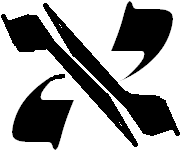There are 18 particle fields, including the graviton; 4 Triplets fields + 4 Essential forces fields, 1 numeral field + 1 Names main field + 1 Essential Cube + 1 special field + 2 constant fields equal 32 in total. There may be other combinations bringing the total to 32. One probable scenario has a separate Name field for 26, for 86, for 21 and for 42.

The other constants may be sub-fields, or they may be derived from the interactions of the 18 particle fields, instead of the other way around where formulas that include the constants give us the energy levels of each particle.

These 32 principle fields represent the primordial Alef(א), or Tree-of-Life, as they come together in the Cube, which may not be a field as we know it, but an Essential scaffolding. These 32 principle fields may balance out with the “The Light(הָאוֹר)” of ordinal value 32.

### 248 Dimensions

Are these 32 fields related to the 248 dimensions of the E8 Lattice? Is the E8 Lattice an expansion or origin of the Essential Cube of Creation? Is the Essential Cube and/or the E8 Lattice the scaffolding for the 32 Fields?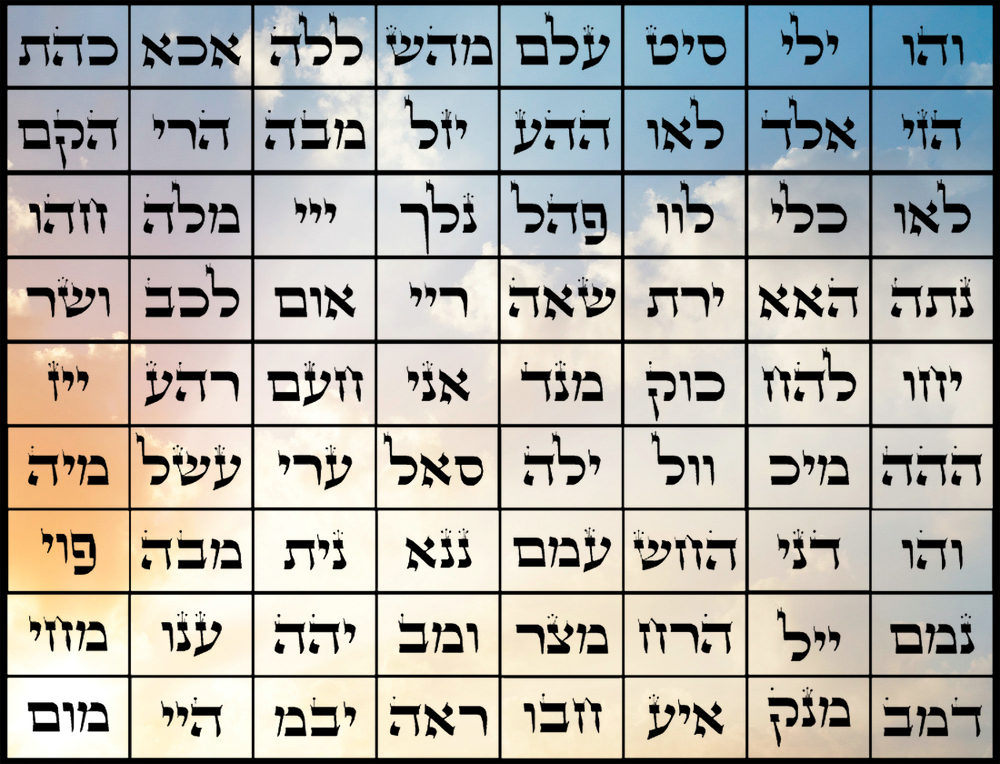The 72 Triplets matrix provides a little clarity of how the schematics of this grand design might work. It is arranged in 9 rows of 8 columns, which is 8 columns of 27 letters each, nicely reimaging the Essential Cube of Creation with its 27 positions and 8 corners. This results in 8 clusters of 27 dimensions, or 216 in total, which when combined with 8 more clusters of 4 each, or the 32 Fields delineated above, gives us (216 + 32) = 248 dimensions and/or Fields of the E8 Lattice subdivided into groups of 8.

These can also be divided into 8 groups of 4 with 8 groups of 27, also giving us (8 x 31) = 248 Fields.  These groups make intuitive sense as the 8 groups of 27 would be iterations of the original Essential Cube of Creation that gave rise to the Flow. It is to be determined the exact complex layout of the E8 Lattice, but under this schematic, every 4 of the 32 delineated Fields could be encased or surrounded by 27 iterations of the original Essential Cube, with each iteration being another dimension, another potential field. Once again, that would be 8 groups of 31.

Those (4 x 27) surrounding fields + the 4 would equal 112, which are not the number of vertices or dimensions in each grouping, just an illustration of their special relationship.

Since each of the 32 delineated Fields is connected to 8 other Fields or dimensional portals, they collectively make 256 or 28 direct connections, and it should be noted that 108, as in the 10 loops or sefirot within the Flow, divided by those 256 connections equals 390,625, the number of words, letters, and verses in the Torah, “The Crowning Heaven (Shama’im H’Keter, 390, 625)” in gematria.

# E8 lattice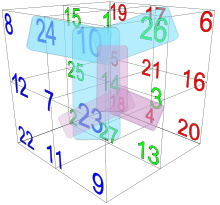The Essential Cube of Creation is very special indeed. Each corner forms a triad with the adjacent cubes (i.e. the number 18 corner point is surrounded by the 2, 4 and 5 points). When we add the opposite corner to it (10 surrounded by 23, 24 and 26) we get 112, as in the 112 Triplets. Every corner triad pair will do that.

The 4 corner triad pairs equal (4 x 112) or 448 and the 8 corners themselves equal a 5th 112. The 8 corner triads are thus 448 and with the 42 that binds them, we have 490 or 7 x 70.

As opposed to the corner triads, there are the tetra central axis, which each average 70 (i.e. 7 surrounded by 12, 24, 23, and 11) each,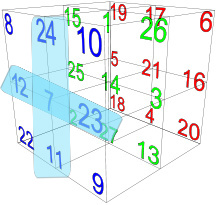making a total of (6 x 70) = 420. Then when we add to it the 3 in the central axes themselves, that total 210, we get (420 + 210) = 630.

It is notable that the central axes total 210 as in the 210 levels and cubits in the Tower or Truth and the Pyramid, and in the 210 years in Egypt (Mizra’im, associated with the firmament found in the center of the Cube) and in the total gematria of the Torah, 21009826.

Altogether, the corner groups and axes groups equal (490 + 630) or 1120.  This is not only (10 x 112), as in one set of the 112 Triplets for every sefira or loop in the Flow, but it is equivalent to the 1120 tetrahedron cells in the E8 lattice.

And let us not forget that 1120 in base 3 is 42 in our world.

Both the subdivisions and the total are divisible by 70, indeed (16 x 70), as in the numerous 70-years blocks of time associated with Biblical history, as discussed in The Divine Calendar, starting with Adam, who lived (100070) years and ending with Israel becoming a nation in 5708 and the event Horizon in 5778.

All this architecture in the Cube happens because of 42, that is evenly split into .333 and .666 as 14 and 28.  Each opposite corner pair equals 28, as does each opposite axis point.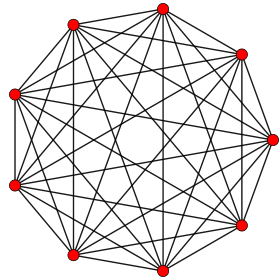As does the power, koach (כח) of the Torah. The total gematria of the collective 304,805 letters in the Torah is 21009826 and the sum of its digits is (2+1+0+0+9+8+2+6) = 28, while the product of those same digits is (2*1*0*0*9*8*2*6) = 1728.  Applying a deeply revealing form of gematria cipher that we have used previously we get an equation that circles us back to the 28 letters of the Torah’s first verse and to the Essential Cube of Creation:

### 4√(P(21009826)/ ∑(21009826))) = 4√(1728/28) = 2.802828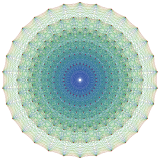Like the 1120 above, the value 1728 is reminiscent of the 17280 8-simplices in the E8 lattice. Likewise, we have the 8-fold expansion of the Essential Cube of Creation from 27 to 216, as in the 2160 8-orthoplexes in the E8 lattice.  The 248-dimensional E8 Lie Group and the E8 lattice is far more complex than we can give it justice. Each point in it is surrounded by 2160 8-orthoplexes and 17280 8-simplices in a 1 to 8 ratio.

As for those 17280 8-simplices, the quantity (17280 x 5) = 86,400, and as previously discussed, the diameter of the sun is 864,000 miles and there are 86,400 seconds in an earth day, which brings up the Hebrew word for day yom, (יום) that is found as one of the 91 select doubled words in the Torah for a value of 112.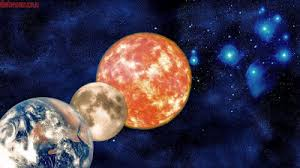Given the harmonics in the design of the universe is it any wonder then that there are 2160 8-orthoplexes in the E8 Lattice and that the diameter of the moon is 2,160 miles?

The Sun, the Moon, and the Earth. Who was this system designed for?

The Torah, the Essential Cube of Creation, the 112 Triplets, and the E8 lattice. Who was this system designed for?

The numeric Field, the Phi and Pi Fields, the 18 Particle Fields, the 4 forces or pressure mediations.  Who was this system designed for?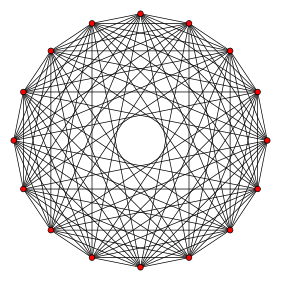Each 8-orthoplex is a regular 8-polytope with 16 vertices, 112 edges, 448 triangle faces, 1120 tetrahedron cells, 1792 5-cells 4-faces, 1792 5-faces, 1024 6-faces, and 256 7-faces.

As for 1792 and 1792 they equal 3584.

As for the 16 vertices, the cosmic reason of their incorporation may have to do with (16 x 70) = 1120, or it may be as simple as (4226) = 16, which is a split darn close to the Phi proportion.  The answer to why 26 or why 42, will no longer be in the math.

## 8 Kings

The verse in Bereshit 36:31, paragraph 72 has multiple deep meanings, including the worlds that existed before our world of tikkun (cause and effect) was created. The thing about the multiple interpretations is that they are all true and do not negate one another. The structure of the E8 lattice and the Essential Cube would necessarily exist before the Torah, Earth, and world of tikkun existed. And there could very well have been both divine and mechanical simulations before our world.  As the Zohar mentions in comments about Vayikra (137-144) and Bereshit 226+ suggests, some of the remnants of those worlds could well coexist with ours even today, giving us explanations for UFOs and aliens that are never spotted leaving or arriving to our planet, but coming out of the sea or hovering over certain areas.

#### The 7 Lower Worlds

While that is interesting, what is germane to our level of analysis lies in the details. The initials (תצנגאאא) of the 7 levels of superimposed prior Earth-like worlds related to the 8 Kings sum to 546, just like the initials of the 10 sefirot/dimensions (כחבחגתנהימ) that have a combined small gematria of 42. It is also like the numerical value for Israel (ישראל) with the kolel for its 5 letters, 546. As we know, according to the Zohar, the distance to Heaven is 500 Parsangs, and the value 46 is that of me’ah (מאה), meaning 100, thus 546 can be cryptically alluding to 500. Moreover, as we pointed out much earlier in this series, 21009826/42019 = 500.00, matching the collective height (500 cubits) of all 10 Gates of the Holy Temple and Temple Mount (excluding the Gate of 42 entrance to the Holy of Holies). It is also equal to both the 23rd Hebrew letter, Caf(ך), of numerical value 500, and the number of years (500) from Abraham’s birth to the reception of the Torah 2448 HC..

Those 7 worlds stretch from Tevel (תבל) of numerical value 432, closest to our world, and the lowest, Ertz (ארץ):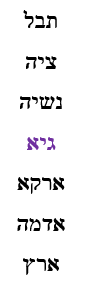What is so interesting about these 7 worlds and how they relate to our universe is that the sum of their initials has a collective ordinal value of 60, as we found concealed in the center of the 42-Letter Name, which leads us to the middle (center) world, (גיא) whose numerical value is 14, as in the central position of the Essential Cube of Creation. Moreover, the numerical value of the initials (תצנ) of the 3 worlds above the 14 world (גיא) is 540 and their ordinal value is 54, the number so connected to the Essential Cube as the 5400 total sum, whose digits totaled 54.

The complete value for the 7 initials, including the kolel, is 613, as in the 613 mitzvot that help us recover the fallen Holy sparks, while the 7 final letters sum to 137, as in Kabbalah, meaning parallel.

Alternatively, using gematria sofit, which Rav Brandwein explained to me elucidated future events, the 7 final letters (לההאאהץ) for the 7 lower parallel worlds equals 947, and as mentioned in The Divine Calendar, the sum value of all the 947 digits in Pi up to the sequence …57781857780… is 4240, as in the value of Moshiach Ben David (424) x 10.

Meanwhile, the gematria for all 7 worlds (432, 105, 365, 14, 302, 50 and 291) is 1559 or (2000 441), Emet, Truth, so reminiscent of the sum of all 10 primordial component letters (דוזיכנסרךן) of the Hebrew Alef-bet, which is 1557, matching the sum of the square roots of the 127 possible combinations of the first 7 words of the Torah. These 10 primordial component letters would also have existed before our world, making them all the more relevant here and their connection to the 7 worlds all the more probable.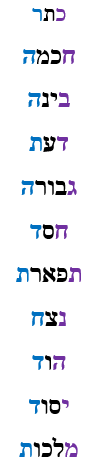The equation of the 7 worlds (432, 105, 365, 14, 302, 50 and 291) = 1559 = (2000 441), Emet, Truth, is significant because the Zohar tells us 2000 years existed before our world and the Torah began and Emet, Truth is the Torah. Now we understand how and why.

Finally, the 24 letters of the 7 parallel pre-worlds are juxtaposed with the 42 letters in the 11 sefirot that include Da’at (דעת).

By the way, the sum numerical value of the 10 sefirot is 2868, or 14 years after King David(דוד), of numerical value 14, was born. In other words, the year (2868 HC) he turned 14. As for the 42 letters of the 11 sefirot, they total 3342. Now, while that is 4 years after the destruction of the First Holy Temple in 3338 HC, it is also the numerical value of the 42-Letter Name, 3701 less the 4th Triplet in it, 359, the value of satan or (3701359) = 3342. Please note that the inclusion of this particular Triplet is as an antidote, made from the venom of the snake.

### “These are the kings who ruled in the land of Edom before any king reigned over the Israelites.” – Bereshit 36:31

One of the deepest and most esoteric revelations is tied to the connections we found with the 72 Triplets and the E8 Lattice. “These are the kings who ruled in the land of Edom before any king reigned over the Israelites.” First, we must understand that the numerical value of the first word in the verse is 42 and that the word Edom(אדום), referring to the lands of Esau and Christianity, has the gematria of 611, that of the “Torah.” The first two words of the verse together “These are the kings” have the gematria of 187, as in the 187 chapters of the Torah, hinting to the full “Torah.”

Second, this verse also contains 1 set of the 91 doubled words in the Torah, (מלך), meaning king. Its gematria is 90, like “water(מים),” which we have already determined refers to the “fields.” Both water and king have a complete gematria of 126, the value of each face and plane in the Essential Cube of Creation, (3 x 42). Together, the doubled words have an ordinal value of 72, strengthening our beliefs in the connections begun above. Their standard gematria is 180, as in the 18 Particle Fields.

Does the story of the 8 Kings refer to the 8 Primal Fields that ruled in the land of the Torah and the E8 Lattice structure that existed before any One(1) = 42  (the central Cube) reigned over the Israelites?

Why would we think it refers to the central 14 -position of the Essential 27 Cubes at the heart of the E8 Lattice. One hint lies in the sum of the square roots of each of the 11 words: 140.027.

Interestingly, like the Torah, the verse begins with 42 and ends with Israel. When does it end?

“Over the Israelites” has a combined gematria of 633, as in “…5778…” at digit number 633.

We exist in a bubble.

# The Beginning and the End

The 11 letters in the Torah’s first and last words together, Bereshit and Israel complete the cycle of the 54 portions.  Numerically, (913 + 541) = 1454, a possible reference to 14 in the center of the 27 positions/fields that total 5400.

Those 4122 recurrences work out to .051541107 of the words in the Torah. Is it about 5% or is it 51, Edom; 515, as in the number of times Moses prayed to G-d to allow him into Israel and bring about Moshiach; 541, as in Israel; and 107 as in the 107th triangular number 5778.

We have just seen how all the various recurrences of the words found in the Torah’s first verse are significant and how they must have been programmed that way from inception.  So while the ordinal value of the first 10 letters of the Torah is 100, as in the 100th prime number, 541, the gematria of the Torah’s last word, Israel, which is found 625 times in the Torah must also be preprogrammed, given that there are precisely 6252 words, letters and verses in the Torah.

Nonetheless, as we saw at the beginning of this series, the splitting of the Alef-bet into 11 odd-even small gematria value pairs sums to 541 and individually those small gematria units total 100. Obviously, also pre-programmed.

By preprogramming, we mean the usage of higher dimensional mathematics that imprints observable circuitry into our world, whether we can comprehend it or not.

Meanwhile, the 11 first letters of the Torah total 112, as in the 112 Triplets that existed before the Torah and that are embedded in it as well. That, coupled with the 11 unique letters in the Torah’s first verse, etc., it becomes revealing that the complete value of the Torah’s first word, Bereshit (בראשית), is (100011), as if “the beginning” lacked something related to 11 for completion.

Bereshit (בראשית) is the first word in the Torah. The root (ראש) of the word for first (ראשון) is in the heart of both Bereshit (בראשית) and Israel (ישראל), last word. It is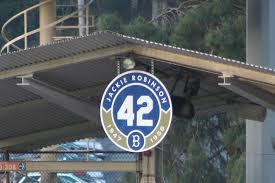also in the higher Name I Am that I Am (אהיה־אשר־אהיה) that is based on 42, as previously explained. The ordinal value of this root (אשר) is 42.

The revealing P/S of this first and last root (אשר) is (60,000/42) = 1428.571428…. and the P/S (60,000/541) rounds out to 111.

The letter Alef (א) is 1, but the two (2) other letters in this root, the Resh(ר) and the Shin(ש), or the 20th and 21st letters in the Alef-bet, have a complete numerical value of (200 + 20 + 300 + 21) = 541, that of Israel, and the spelled out value for 2 iterations of (200 + 300 + 510 + 1010) = 2020, as in 2020 CE.

Separating the root of the word splits the complete value of the Torah’s first word Bereshit (בראשית) into, 541 and 448, or Israel and 4 x 112, as in the 112 Triplets that surround the Essential Cube of 42.Higher dimensional math reveals that the sum of the digits from 1 – 20 is 210 for the letter Resh(ר) and from 1 – 21 is 231 for the letter Shin(ש). Together (210 + 231) = 441, Emet, Truth. Add 100 for the 100th prime and we get 541, Israel.

“G-d named the firmament (רקיע) Heaven (שמים)”

Then through the heart of the 42-Letter Name, we find that the 21st letter in the Name is Resh(ר), the 20th letter of the Alef-bet. While (21 x 20) = 420, the product of their values (200 x 300) = 60,000, as in the letter Samech (ס) of numerical value of 60. It is the 1833 Torah occurrences of that letter Samech (ס) that align with the numerical value of the first 21 letters at the midpoint of the 42 Letter Name.

While mathematics opens our eyes and tells us where to look, the answer to why 42, will no longer be in the math.

Ezra

For anyone that has not been following this series from its inception, you can find the entire work here.